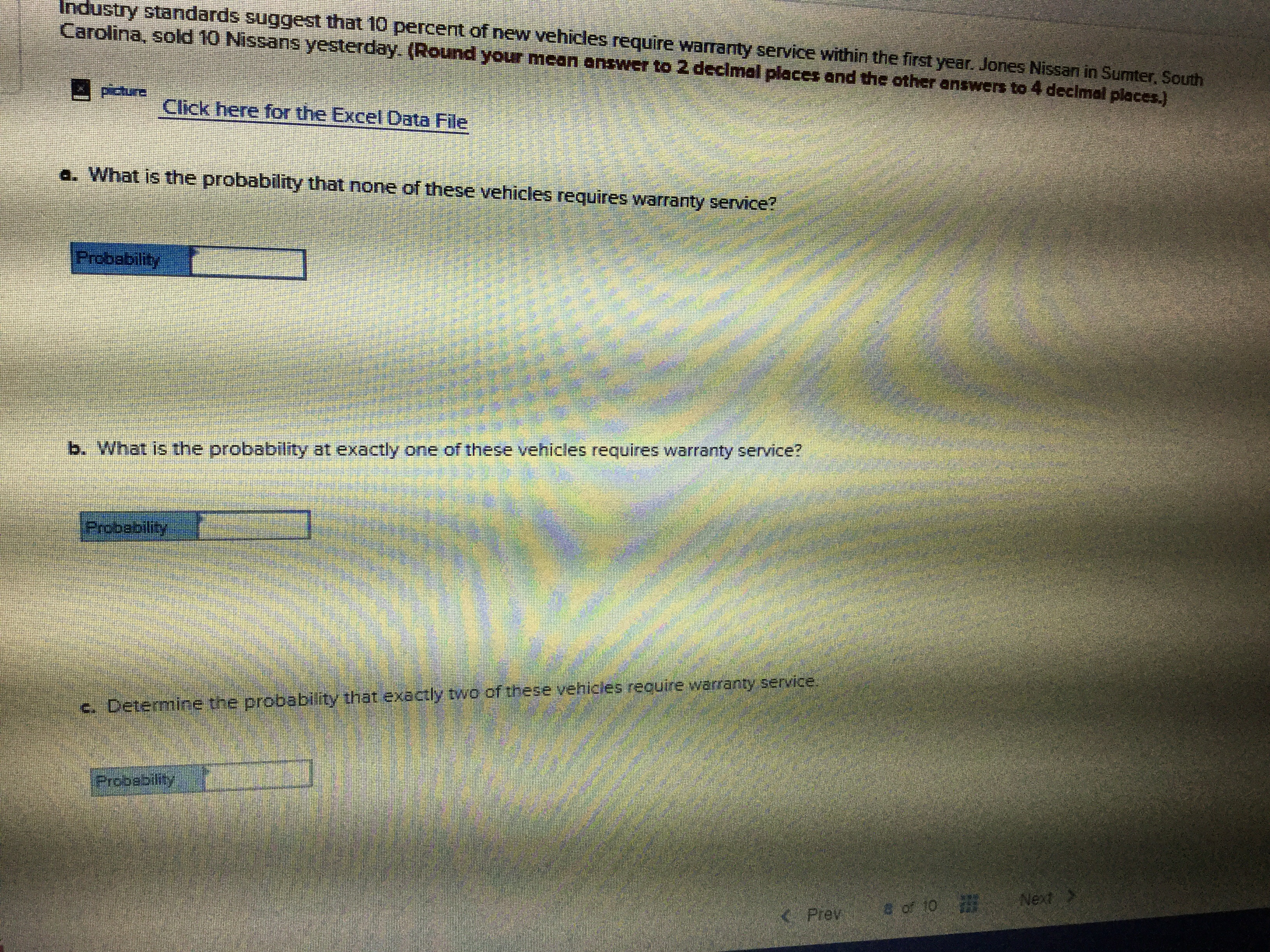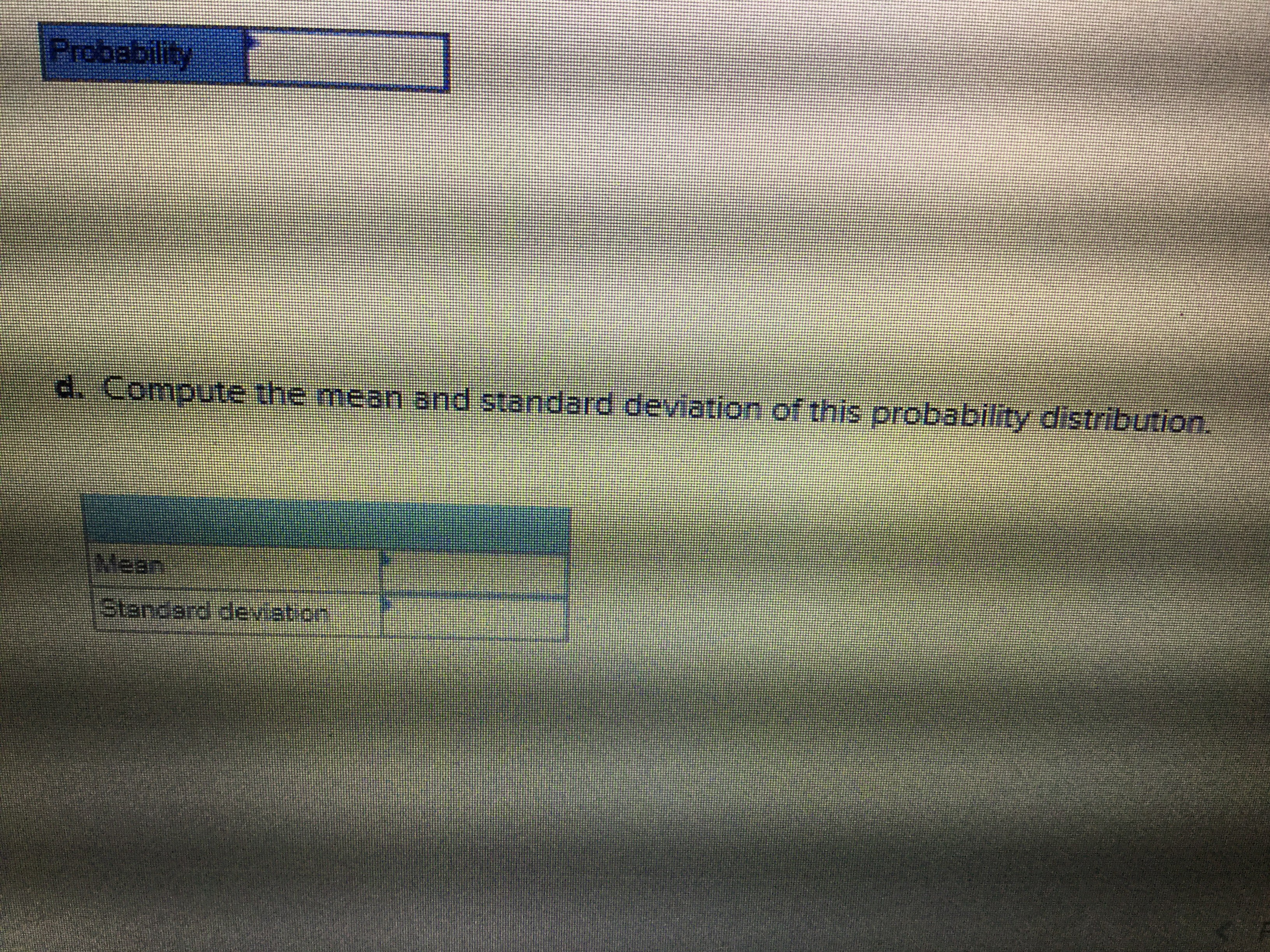# Industry standards suggest that 10 percert of new vehicles require warranity service within the first year. Jones Nissan in Sumter, SouthCarolina, sold 10 Nissans yesterday (Round your mean answer to 2 decimal places and the other answers to 4 decimal places.)ביחאב3-Click here for the Excel Data Filea. What is the probability that none of these vehicles requires warranty service?Probabilityb. What is the probability at exactly one of these vehicles requires warranty service?Probabilityof these vehices require warranty servicec. Detemine the probability that exactly twoProbabilityNext >a of 10Prev Probabilityd. Compute the mean and standard deviotion of this probability distribution.eanStandard deviation

Question
21 viewshelp_outlineImage TranscriptioncloseIndustry standards suggest that 10 percert of new vehicles require warranity service within the first year. Jones Nissan in Sumter, South Carolina, sold 10 Nissans yesterday (Round your mean answer to 2 decimal places and the other answers to 4 decimal places.) ביחאב 3- Click here for the Excel Data File a. What is the probability that none of these vehicles requires warranty service? Probability b. What is the probability at exactly one of these vehicles requires warranty service? Probability of these vehices require warranty service c. Detemine the probability that exactly two Probability Next > a of 10 Prev fullscreenhelp_outlineImage TranscriptioncloseProbability d. Compute the mean and standard deviotion of this probability distribution. ean Standard deviation fullscreen
check_circle

Step 1

Step 1:

Consider a random variable X which, follows binomial distribution, indicates the number of vehicles requires warranty.

According to the provided data, the number of trials, n is 10 and the probability, p of vehicles requires warranty is 0.10.

The binomial distribution formula is used here,

Step 2

a. The probability that none of these vehicles requires warranty service is,

Step 3

b. The probability at exactly one of these v...

### Want to see the full answer?

See Solution

#### Want to see this answer and more?

Solutions are written by subject experts who are available 24/7. Questions are typically answered within 1 hour.*

See Solution
*Response times may vary by subject and question.
Tagged in

### Statistics